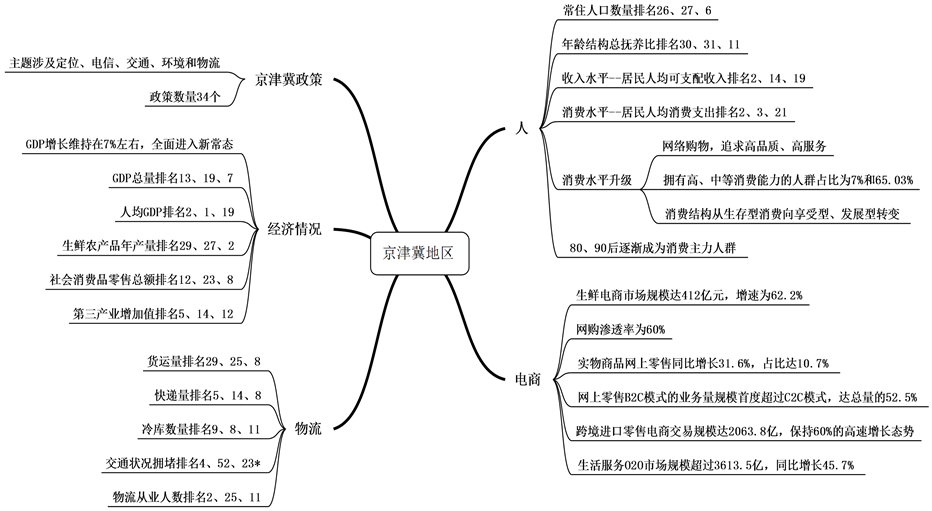﻿ 基于大数据的京津冀农产品物流需求预测分析 Prediction and Analysis of Agricultural Products Logistics Demand in Beijing, Tianjin and Hebei Based on Big Data

Operations Research and Fuzziology
Vol.08 No.03(2018), Article ID:26461,10 pages
10.12677/ORF.2018.83016

Prediction and Analysis of Agricultural Products Logistics Demand in Beijing, Tianjin and Hebei Based on Big Data

Songmiao Li

Beijing Wuzi University, BeijingReceived: Jul. 27th, 2018; accepted: Aug. 9th, 2018; published: Aug. 16th, 2018ABSTRACT

The national strategy of Beijing-Tianjin-Hebei coordinated strategy brings opportunities for development in the logistics market. People are paying more and more attention to the safety and health of foodstuffs. At the same time, with the upgrade of people's consumption, the widespread concern about the quality and freshness of agricultural products poses a great challenge to the logistics industry. Based on the demand of agricultural products logistics, this paper establishes the index system of agricultural products logistics, designs the forecasting model based on big data and factor analysis and multiple linear regression, predicts and analyzes the logistics demand of agricultural products by using the model. It is found that the key factors influencing the logistics demand in Beijing, Tianjin and Hebei are the macro economy and freight volume, and put forward the suggestions that the Beijing-Tianjin-Hebei region should be developed according to the regional positioning, vigorously develop the logistics and communication infrastructure and provide high-quality logistics services.

Keywords:Beijing-Tianjin-Hebei Region, Agricultural Product Logistics, Big Data Forecast, SPSSCopyright © 2018 by author and Hans Publishers Inc.1. 引言

2. 研究方法和数据选择

2.1. 研究方法

2.1.1. 大数据

2.1.2. 因子分析方法

2.1.3. 多元回归分析

2.2. 数据选择

3. 模型的建立和农产品物流需求预测

3.1. 模型的建立

${F}_{Pi}=\sum _{j=1}^{k}{W}_{Pj}{X}_{ji}$注：*表示河北省进入排名的有4个城市，此处选择最靠前的资料来源：国家统计局和相关行业报告

Figure 1. Big data portraits of the Beijing-Tianjin-Hebei regionTable 1. Agricultural products logistics demand influencing factor index system

1) 设观察到的随机向量为 $X=\left({x}_{1},{x}_{2},\cdots ,{x}_{p}\right)$ ，不可观察向量为 $F=\left({F}_{1},{F}_{2},\cdots ,{F}_{p}\right)$ ，则有：

$\left\{\begin{array}{l}{x}_{1}={a}_{11}{F}_{1}+{a}_{12}{F}_{2}+\cdots +{a}_{1m}{F}_{m}+{\epsilon }_{1}\\ {x}_{2}={a}_{21}{F}_{1}+{a}_{22}{F}_{2}+\cdots +{a}_{2m}{F}_{m}+{\epsilon }_{2}\\ \text{\hspace{0.17em}}\text{\hspace{0.17em}}\text{\hspace{0.17em}}\text{\hspace{0.17em}}\text{\hspace{0.17em}}⋮\\ {x}_{p}={a}_{p1}{F}_{1}+{a}_{p2}{F}_{2}+\cdots +{a}_{pm}{F}_{m}+{\epsilon }_{p}\end{array}$

2) 如果因子荷载矩阵不能对主因子进行解释时，需要进一步做因子旋转，使影响因子具有高负载，使因子更加具有合理的解释。

3) 计算因子得分数学模型：

${F}_{j}={b}_{i1}{X}_{1}+{b}_{i2}{X}_{2}+\cdots +{b}_{in}{X}_{n}$

4) 利用SPSS19.0进行多元线性回归分析，建立冷链物流需求预测的多元线性回归模型，并对模型进行各种相关检验，即：

$Y={q}_{0}+{q}_{1}{F}_{1}+{q}_{2}{F}_{2}+\cdots +{q}_{p}{F}_{p}+\epsilon$

3.1.1. KMO和Bartlett的检验判断是否适合因子分析

3.1.2. 提取因子

3.1.3. 命名性解释

3.1.4. 计算因子得分

$\begin{array}{c}{F}_{1}={x}_{1}*0.130+{x}_{2}*0.130+{x}_{3}*0.128+{x}_{4}*0.129+{x}_{5}*0.131\\ \text{\hspace{0.17em}}\text{\hspace{0.17em}}+{x}_{6}*0.129+{x}_{7}*0.034+{x}_{8}*0.129+{x}_{9}*0.124\end{array}$

$\begin{array}{c}{F}_{2}=-{x}_{1}*0.013+{x}_{2}*0.077-{x}_{3}*0.026-{x}_{4}*0.010+{x}_{5}*0.003\\ \text{\hspace{0.17em}}\text{\hspace{0.17em}}+{x}_{6}*0.040+{x}_{7}*0.946+{x}_{8}*0.179-{x}_{9}*0.125\end{array}$

3.1.5. 建立回归方程

3.2. 农产品物流需求预测

$y=727.789-0.017{F}_{1}$Table 9. Regression equation variable of BeijingTable 10. Forecast of agricultural products logistics demand in Beijing from 2006 to 2015Table 12. Forecast of agricultural products logistics demand in Beijing from 2016 to 2020Table 13. Forecast of agricultural products logistics demand in Beijing-Tianjin-Hebei region from 2016 to 2020

4. 预测结果与建议

4.1. 结果分析

4.2. 京津冀农产品物流发展建议

4.2.1. 遵循地区定位发展经济

4.2.2. 大力发展物流和通信基础设施

4.2.3. 提供高质量的物流服务

Prediction and Analysis of Agricultural Products Logistics Demand in Beijing, Tianjin and Hebei Based on Big Data[J]. 运筹与模糊学, 2018, 08(03): 127-136. https://doi.org/10.12677/ORF.2018.83016

1. 1. 洪玉兰, 肖其荣. 浅谈我国农产品冷链物流存在的问题及发展建议[J]. 物流科技, 2010, 33(9): 42-44.

2. 2. 杨文凤. 西藏农产品物流需求研究[D]: [硕士学位论文]. 杨凌: 西北农林科技大学, 2012.

3. 3. 李合龙, 李妍, 郑雪仪. SPSS统计学实验教程[M]. 北京: 清华大学出版社, 2015.

4. 4. 刘宇, 吴迎学, 党文峰. 基于多元线性回归的区域物流需求预测研究[J]. 物流技术, 2014(3): 52-54.

5. 5. 邱慧, 黄解宇, 董亚兰. 基于灰色系统模型的山西省物流需求预测分析[J]. 数学的实践与认识, 2016, 46(13): 66-70.

6. 6. 李夏培. 基于灰色线性组合模型的农产品物流需求预测[J]. 北京交通大学学报(社会科学版), 2017, 16(1): 120-126.

7. 7. 易观智库. 2016年主流消费人群六大标签与互联网行业变化分析[EB/OL]. http://www.useit.com.cn/thread-11505-1-1.html, 2016-03-03.

8. 8. 高德地图. 2017年第一季度中国主要城市交通分析报告[R]. 北京: 高德软件有限公司, 交通运输部科学研究院, 清华大学–戴姆勒可持续交通研究中心, 阿里云, 2017.

9. 9. 智研咨询. 2017~2022年中国生鲜电商行业市场现状分析及投资前景预测报告[R]. 北京: 智研咨询集团, 2017.

10. 10. 中国新闻网. 官方明确京津冀功能定位北京担当“四个中心” [EB/OL]. http://www.chinanews.com/gn/2015/08-23/7484049.shtm, 2016-10-26.